Fit Analyses

## Nonparametric Local Polynomial Smoother

The kernel estimator fits a local mean at each point x and thus cannot even estimate a line without bias (Cleveland, Cleveland, Devlin and Grosse 1988). An estimator based on locally-weighted regression lines or locally-weighted quadratic polynomials may give more satisfactory results.

A local polynomial smoother fits a locally-weighted regression at each point x to produce the estimate at x. Different types of regression and weight functions are used in the estimation.

SAS/INSIGHT software provides the following three types of regression: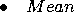a locally-weighted mean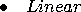a locally-weighted regression line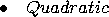a locally-weighted quadratic polynomial regression

The weights are derived from a single function that is independent of the design
where K0 is a weight function and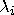is the local bandwidth at xi.

SAS/INSIGHT software uses the following weight functions: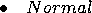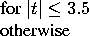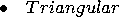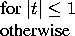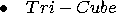Note The normal weight function is proportional to a truncated normal density function.

SAS/INSIGHT software provides two methods to compute the local bandwidth.The loess estimator (Cleveland 1979; Cleveland, Devlin and Grosse 1988) evaluatesbased on the furthest distance from k nearest neighbors. A fixed bandwidth local polynomial estimator uses a constant bandwidth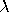at each xi.

For a loess estimator, you select k nearest neighbors by specifying a positive constant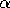.For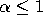, k is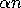truncated to an integer, where n is the number of observations. For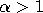, k is set to n.

The local bandwidthis then computed as
where d(k)( xi) is the furthest distance from xi to its k nearest neighbors.Note For, the local bandwidthis a function of k and thus a step function of.

For a fixed bandwidth local polynomial estimator, you select a bandwidthby specifying c in the formula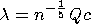where Q is the sample interquartile range of the explanatory variable and n is the sample size. This formulation makes c independent of the units of X.Note A fixed bandwidth local mean estimator is equivalent to a kernel smoother.

By default, SAS/INSIGHT software divides the range of the explanatory variable into 128 evenly spaced intervals, then it fits locally-weighted regressions on this grid. A small value of c ormay give the local polynomial fit to the data points near the grid points only and may not apply to the remaining points.

For a data point xi that lies between two grid points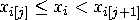, the predicted value is the weighted average of the two predicted values at the two nearest grid points: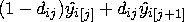where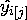and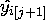are the predicted values at the two nearest grid points and
dij = [(xi- xi[j])/(xi[j+1]- xi[j] )]

A similar algorithm is used to compute the degrees of freedom of a local polynomial estimate,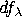= trace(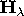). The ith diagonal element of the matrixis

(1- dij) hi[j] + dij hi[j+1]
where hi[j] and hi[j+1] are the ith diagonal elements of the projection matrices of the two regression fits.

After choosing Curves:Loess from the menu, you specify a loess fit in the Loess Fit dialog.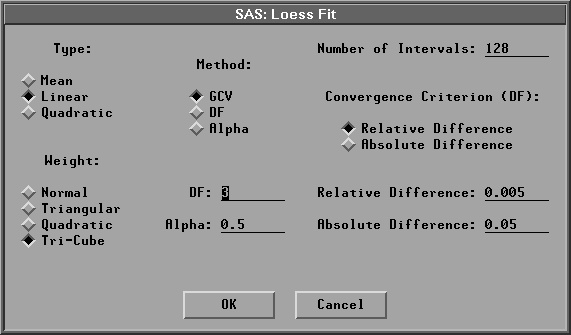Figure 39.44: Loess Fit Dialog

In the dialog, you can specify the number of intervals, the regression type, the weight function, and the method for choosing the smoothing parameter. The default Type:Linear uses a linear regression, Weight:Tri-Cube uses a tri-cube weight function, and Method:GCV uses anvalue that minimizes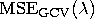.

Figure 39.45 illustrates loess estimates with Type=Linear, Weight=Tri-Cube, andvalues of 0.0930 (the GCV value) and 0.7795 (DF=3). Use the slider to change thevalue of the loess fit.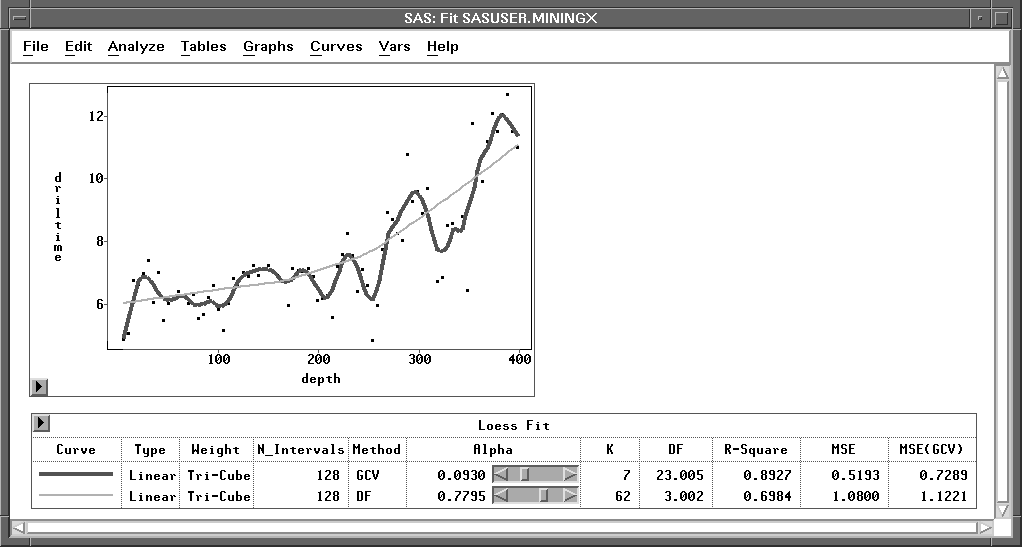Figure 39.45: Loess Estimates

The loess degrees of freedom is a function of local bandwidth.For,is a step function ofand thus the loess df is a step function of.The convergence criterion applies only when the specified df is less than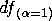,the loess df for.When the specified df is greater than, SAS/INSIGHT software uses thevalue that has its df closest to the specified df.

Similarly, you can choose Curves:Local Polynomial, Fixed Bandwidth from the menu to specify a fixed bandwidth local polynomial fit.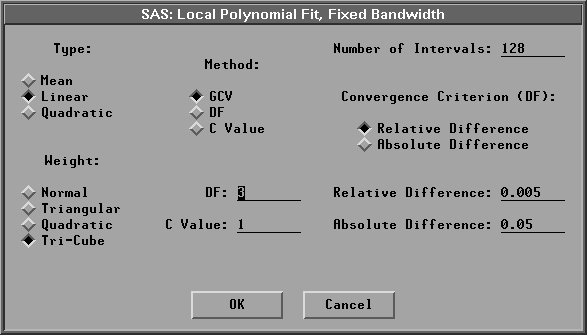Figure 39.46: Fixed Bandwidth Local Polynomial Fit Dialog

Figure 39.47 illustrates fixed bandwidth local polynomial estimates with Type=Linear, Weight=Tri-Cube, and c values of 0.2026 (the GCV value) and 2.6505 (DF=3). Use the slider to change the c value of the local polynomial fit.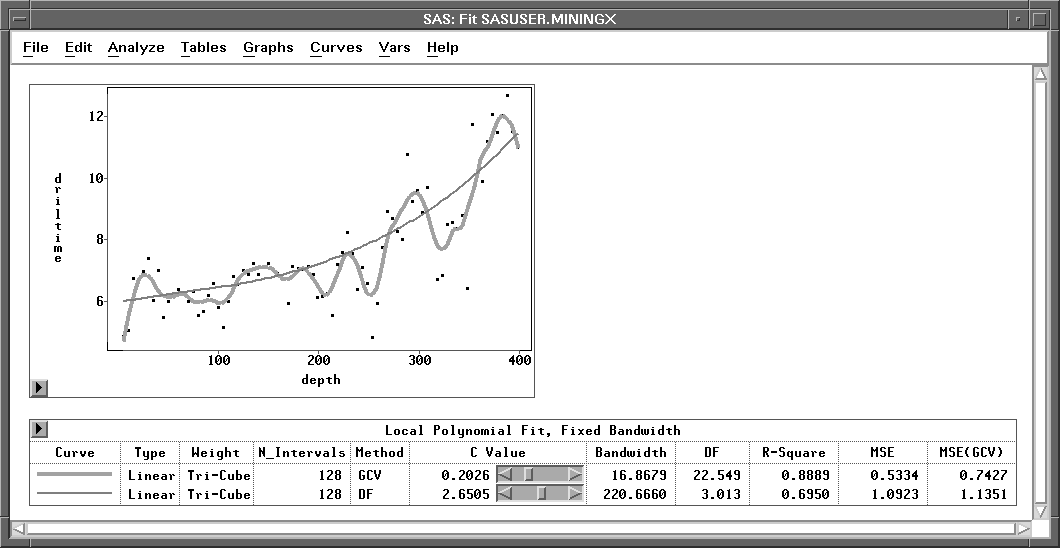Figure 39.47: Fixed Bandwidth Local Polynomial Estimates